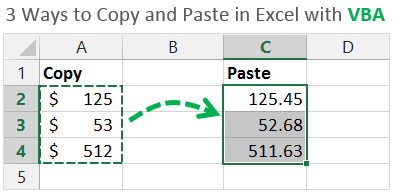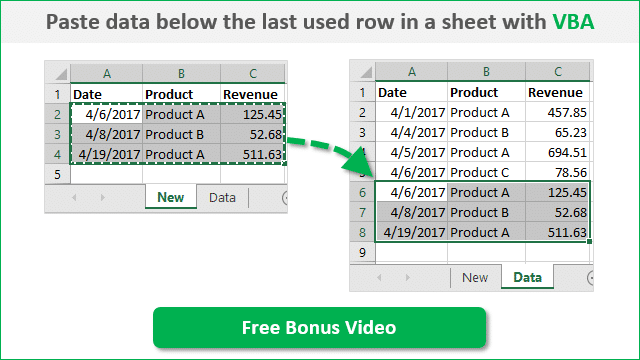Bottom line: Learn 3 different ways to copy and paste cells or ranges in Excel with VBA Macros.  This is a 3-part video series and you can also download the file that contains the code.

Skill level: Beginner## Copy & Paste: The Most Common Excel Action

Copy and paste is probably one of the most common actions you take in Excel.  It's also one of the most common tasks we automate when writing macros.

There are a few different ways to accomplish this task, and the macro recorder doesn't always give you the most efficient VBA code.

In the following three videos I explain:

• The most efficient method for a simple copy and paste in VBA.
• The easiest way to paste values.
• How to use the PasteSpecial method for other paste types.

You can download the file I use in these videos below.  The code is also available at the bottom of the page.VBA Copy Paste Methods.xlsm (91.7 KB)

## Video #1: The Simple Copy Paste Method

You can watch the playlist that includes all 3 videos at the top of this page.

## VBA Code for the Copy & Paste MethodsVBA Copy Paste Methods.xlsm (91.7 KB)

```'3 Methods to Copy & Paste with VBA
'Source: https://www.excelcampus.com/vba/copy-paste-cells-vba-macros/
'Author: Jon Acampora

Sub Range_Copy_Examples()
'Use the Range.Copy method for a simple copy/paste

'The Range.Copy Method - Copy & Paste with 1 line
Range("A1").Copy Range("C1")
Range("A1:A3").Copy Range("D1:D3")
Range("A1:A3").Copy Range("D1")

'Range.Copy to other worksheets
Worksheets("Sheet1").Range("A1").Copy Worksheets("Sheet2").Range("A1")

'Range.Copy to other workbooks
Workbooks("Book1.xlsx").Worksheets("Sheet1").Range("A1").Copy _
Workbooks("Book2.xlsx").Worksheets("Sheet1").Range("A1")

End Sub

Sub Paste_Values_Examples()
'Set the cells' values equal to another to paste values

'Set a cell's value equal to another cell's value
Range("C1").Value = Range("A1").Value
Range("D1:D3").Value = Range("A1:A3").Value

'Set values between worksheets
Worksheets("Sheet2").Range("A1").Value = Worksheets("Sheet1").Range("A1").Value

'Set values between workbooks
Workbooks("Book2.xlsx").Worksheets("Sheet1").Range("A1").Value = _
Workbooks("Book1.xlsx").Worksheets("Sheet1").Range("A1").Value

End Sub

Sub PasteSpecial_Examples()
'Use the Range.PasteSpecial method for other paste types

'Copy and PasteSpecial a Range
Range("A1").Copy
Range("A3").PasteSpecial Paste:=xlPasteFormats

'Copy and PasteSpecial a between worksheets
Worksheets("Sheet1").Range("A2").Copy
Worksheets("Sheet2").Range("A2").PasteSpecial Paste:=xlPasteFormulas

'Copy and PasteSpecial between workbooks
Workbooks("Book1.xlsx").Worksheets("Sheet1").Range("A1").Copy
Workbooks("Book2.xlsx").Worksheets("Sheet1").Range("A1").PasteSpecial Paste:=xlPasteFormats

'Disable marching ants around copied range
Application.CutCopyMode = False

End Sub```

## Paste Data Below the Last Used Row

One of the most common questions I get about copying and pasting with VBA is, how do I paste to the bottom of a range that is constantly changing?  I first want to find the last row of data, then copy & paste below it.

To answer this question, I created a free training video on how to paste data below the last used row in a sheet with VBA.  Can I send you the video?  Please click the image below to get the video.## Free Training on Macros & VBA

The 3 videos above are from my VBA Pro Course.  If you want to learn more about macros and VBA then checkout my free 3-part video training series.

I will also send you info on the VBA Pro Course, that will take you from beginner to expert.  Click the link below to get instant access.

Free Training on Macros & VBA

•Najar says:

Hi jon,
Thanks for your this tutorial, but i need a urgent help if possible answer to me.

copy and paste only filter cells from one excel to other excel using VBA
excel-vba
I’m stuck with a problem when I try to copy filtered cells from one excel file and paste it onto another file. I am using a macro call to get data where I try to fix this given code. It is not working when I try to copy visible cells which i filtered.
Private Sub CommandButton1_Click()

Dim lrCD As Long
Dim fNameAndPath As Variant
Dim WB As Workbook
Dim SourceWB As Workbook
Dim WS As Worksheet
Dim ASheet As Worksheet
fNameAndPath = Application.GetOpenFilename(FileFilter:=”Excel Files (*.xlsx), *.xlsx”, Title:=”Seleziona il file da aprire”)

‘Sets the variables:
Set WB = ActiveWorkbook
Set ASheet = ActiveSheet
Set SourceWB = Workbooks.Open(fNameAndPath) ‘Modify to match

‘Copies each sheet of the SourceWB to the end of original wb:
For Each WS In SourceWB.Worksheets
WS.Copy after:=WB.Sheets(WB.Sheets.Count)
Next WS

SourceWB.Close savechanges:=False
Set WS = Nothing
Set SourceWB = Nothing

WB.Activate
ASheet.Select

Set ASheet = Nothing
Set WB = Nothing

Application.EnableEvents = True
lastrow = Worksheets(4).Cells(Rows.Count, 1).SpecialCells(xlCellTypeVisible).End(xlUp).Row

For i = 3 To lastrow
Worksheets(4).Cells(i, 16).SpecialCells(xlCellTypeVisible).Copy
erow = Worksheets(“CFF”).Cells(Rows.Count, 1).SpecialCells(xlCellTypeVisible).End(xlUp).Row
Worksheets(4).PasteSpecial xlPasteValues = Worksheets(“CFF”).Cells(erow + 1, 2)
Worksheets(4).Cells(i, 16).SpecialCells(xlCellTypeVisible).Copy
Worksheets(4).PasteSpecial xlPasteValues = Worksheets(“CFF”).Cells(erow + 1, 3)
Worksheets(4).Cells(i, 15).SpecialCells(xlCellTypeVisible).Copy
Worksheets(4).PasteSpecial xlPasteValues = Worksheets(“CFF”).Cells(erow + 1, 4)
Worksheets(4).Cells(i, 12).SpecialCells(xlCellTypeVisible).Copy
Worksheets(4).PasteSpecial xlPasteValues = Worksheets(“CFF”).Cells(erow + 1, 5)
Worksheets(4).Cells(i, 13).SpecialCells(xlCellTypeVisible).Copy
Worksheets(4).PasteSpecial xlPasteValues = Worksheets(“CFF”).Cells(erow + 1, 6)
Worksheets(4).Cells(i, 18).SpecialCells(xlCellTypeVisible).Copy
Worksheets(4).PasteSpecial xlPasteValues = Worksheets(“CFF”).Cells(erow + 1, 1)
Next i

Application.CutCopyMode = False

Sheets(4).Select
ActiveWindow.SelectedSheets.Delete

Sheets(2).Select

End Sub

•shaik riyaz says:

SUPER

•Shubham Singh Thakur says:

I wanted to maintain a formatting of one of my records. In which I want to copy formats of certain cells to no. of times the user enters this will reduce the time of formatting ( which is a lot for me). Can you please make a video on that plz?

•Shovan Saha says:

Dim lastRow1 As Long, erow1 As Long
lastRow1 = Worksheets(“WO_SendM”).Cells(Rows.Count, 1).End(xlUp).Row
For i = 3 To lastRow1

If Worksheets(“WO_SendM”).Cells(i, 9).Value = Me.Label164.Caption Then
Worksheets(“WO_SendM”).Cells(i, 4).Copy
Worksheets(“WO_SendM”).Cells(i, 5).Copy

erow1 = Worksheets(“WO_Ledger”).Cells(Rows.Count, 18).End(xlUp).Row
Worksheets(“WO_SendM”).Paste Destination:=Worksheets(“WO_Ledger”).Cells(erow1 + 1, 18)
End If
Next i

Is it possible to get two cell value 4 and 5 in another cell?

•Clara Arganaraz says:

Hi John,

With a macro I am copying the last row of a table and pasting below as PasteFormulas, but when I do this, it consider the row as a ” total row” of the table. I do it in two different tables and in one it paste it okay but in the other one it considers it as a “Total Row” of the table.

Can you help me?

Many thanks!

•jeannie says:

will this work to copy a formula and use it on next line?

•Munna says:

i have 300+ excel workbooks.
my requirement is copy specific data from all workbooks to another new workbook though VBA, can this possible,

•Prabhat Kumar says:

Yes it is possible
Sub Copy()
Dim Sheet1 as worksheet
Dim sheet2 as worksheet
Dim book1 as workbook
Dim book2 as workbook

Workbooks(“Book1.xlsx”).Worksheets(“Sheet1”).Range(“A1”).Copy
Workbooks(“Book2.xlsx”).Worksheets(“Sheet1”).Range(“A1”).PasteSpecial Paste:=xlPasteFormats

End sub

•nabiuddin says:

nabiuddin@gmail.com
kindly share it

•Shashaank H K says:

Can you tell me how to Cells from Sheets present in 4 or more Workbooks to one Final Workbook?

Thank You.

•Tim says:

I have a workbook with a few tabs in it. I want to copy a cell with a formula in it from one worksheet and paste it into a cell in a different worksheet. When I use “PasteSpecial Paste:=xlPasteFormulas” it turns the formula cell references into REF# errors. For example:

LEN(A2)-LEN(SUBSTITUTE(A2,” “,””))=0
becomes
LEN(REF#)-LEN(SUBSTITUTE(REF#,” “,””))=0

It’s pasting the formula into the destination worksheet, but it must think the cell reference is still related to the source worksheet…or something like that. Do you know what could be causing this? Thank you!

•Kari says:

I am trying to write VBA for the below.
If the value in column AA is =”-” and the value in column AC is \$0.00 then copy the value in column X to Column AA. My data set is ever changing so I can not set specific ranges and it may be the case where I don’t have data the satisfies the above criteria.

•paul says:

Hello, nice writeup. One quick question- Instead of copying an orderly range of cells from one column, how may we copy multiple single cells & paste them into an orderly horizontal range?

For example copying B2, F9, and Q11 yet pasting them into A1:C1
Thank you!

•Deepak says:

Hi Jon,

I have a crunched data in one workbook and wanted paste it in other by using macro. Also the number of line items changes every day (increase or decrease), So help me to copy and pasting the data in main template without number mismatch.

•chris says:

OK I’m having a little trouble making a macro for a project at work. What I need help with: i have one work book and user form on sheet one to fill in information and this user form i have a button that should copy range of cells on sheet2 (S6:S9) to the (L6:L9)(all of this is on sheet2 and the samr thing should happen sheet3 to sheet9. If i could get some help i would be grateful

•alam says:

•Diego Rivera says:

Hi,

I need your support, I’m creating a workbook for daily report, and I’m stuck in the summary worksheet, in the VBA I don’t know how to link the information of the new report to be copied in the summary.

Sub GENERAR_REPORTE()

Sheets(“REPORTE DIARIO”).Copy After:=Sheets(ActiveWorkbook.Sheets.Count)
ActiveSheet.Shapes.Range(Array(“Button 1”)).Delete
ActiveSheet.Name = Range(“P7”).Value
Sheets(“REPORTE DIARIO”).Delete

End Sub

Sub REGISTRAR_REPORTE()

Worksheets(“SUMMARY”).Range(“A6”).Value = ActiveSheet.Name = Range(“P7”).Value.Range(“P7”).Value
Worksheets(“SUMMARY”).Range(“G6”).Value = ActiveSheet.Name = Range(“P7”).Value.Range(“B15”).Value
Worksheets(“SUMMARY”).Range(“H6”).Value = ActiveSheet.Name = Range(“P7”).Value.Range(“D15”).Value
Worksheets(“SUMMARY”).Range(“I6”).Value = ActiveSheet.Name = Range(“P7”).Value.Range(“F15”).Value
Worksheets(“SUMMARY”).Range(“J6”).Value = Worksheets(“REPORTE DIARIO”).Range(“H15”).Value
Worksheets(“SUMMARY”).Range(“K6”).Value = Worksheets(“REPORTE DIARIO”).Range(“J15”).Value
Worksheets(“SUMMARY”).Range(“L6”).Value = Worksheets(“REPORTE DIARIO”).Range(“L15”).Value
Worksheets(“SUMMARY”).Range(“M6”).Value = Worksheets(“REPORTE DIARIO”).Range(“N15”).Value
Worksheets(“SUMMARY”).Range(“N6”).Value = Worksheets(“REPORTE DIARIO”).Range(“P15”).Value

•joe78man says:

Windows(“incident.xlsx”).Activate
Range(“A:U”).Select ‘this trows the error: application-defined or object-deferred error

•Catherine says:

Hi, I have two workbooks opened (both can be different names) and I want to copy a Range from Workbook1 SheetX (unkown/active) to Workbook2 (where I know the Sheet name)
I even want the command to open the worksheet from a directory too. Do you have any suggestions on how to do this? Hope to hear from you. Many thanks.

•Erika says:

Hi,
I have two workbooks opened (both can be different names) and I want to copy a Range from Workbook1 SheetX (unkown/active) to Workbook2 (where I know the Sheet name)
Do you have any suggestions on how to do this?
Thanks,
Erika

•BKS says:

you need to take work under one variable than activate the workbook and select particular sheet. You have to write code to copy paste in active workbook if you have any specific sheet than write sheet name.

Generic filters
Exact matches only
Filter by Custom Post TypeLearn 10 great Excel techniques that will wow your boss and make your co-workers say, "how did you do that??"